## How to Calculate and Solve for Period | Motion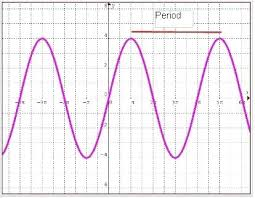The image above represents period.

To compute for period, two essential parameters are needed and these parameters are length (l) and acceleration due to gravity (g).

The formula for calculating period:

T = 2π(√(l / g))

Where;

T = Period
l = Length
g = Acceleration due to gravity

Let’s solve an example;
Find the period when the length is 4 and the acceleration due to gravity is 9.8.

This implies that;

l = Length = 4
g = Acceleration due to gravity = 9.8

T = 2π(√(l / g))
T = 2π(√(4 / 9.8))
T = 2π(√(0.408))
T = 2π(0.6388)
T = 6.28 x 0.6388
T = 4.014

Therefore, the period is 4.014 s.

Calculating the Length when the Period and the Acceleration due to Gravity is Given.

l = (T / )2 x g

Where;

l = Length
T = Period
g = Acceleration due to gravity

Let’s solve an example;
Find the length when the period is 28 and the acceleration due to gravity is 9.8.

This implies that;

T = Period = 28
g = Acceleration due to gravity = 9.8

l = (T / )2 x g
l = (28 / 6.28)2 x 9.8
l = (4.458)2 x 9.8
l = 19.87 x 9.8
l = 194.7

Therefore, the length is 194.7.

## How to Calculate and Solve for TEX | Polymer & Textile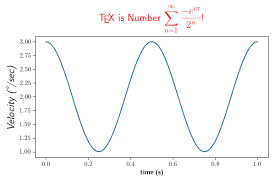The image above represents TEX.

To compute for TEX, two essential parameters are needed and these parameters are mass (M) and length (L).

The formula for calculating TEX:

TEX = M/L x 1000

Where:

TEX = TEX
M = Mass
L = Length

Let’s solve an example;
Find the TEX when the mass is 8 and the length is 14.

This implies that;

M = Mass = 8
L = Length = 14

TEX = M/L x 1000
TEX = 8/14 x 1000
TEX = 0.57 x 1000
TEX = 571.4

Therefore, the TEX is 571.4.

Calculating the Mass when the TEX and the Length is Given.

M = TEX x L / 1000

Where:

M = Mass
TEX = TEX
L = Length

Let’s solve an example;
Find the mass when the TEX is 40 and the length is 8.

This implies that;

TEX = TEX = 40
L = Length = 8

M = TEX x L / 1000
M = 40 x 8 / 1000
M = 320 / 1000
M = 0.32

Therefore, the mass is 0.32.

## How to Calculate and Solve for Denier | Polymer & Textile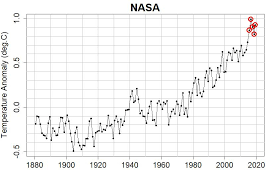The image above represents denier.

To compute for denier, two essential parameters are needed and these parameters are mass (M) and length (L).

The formula for calculating denier:

Denier = M/L x 9000

Where;

D = Denier
M = Mass
L = Length

Let’s solve an example;
Find the denier with a mass of 24 and a length of 28.

This implies that;

M = Mass = 24
L = Length = 28

Denier = M/L x 9000
Denier = 24/28 x 9000
Denier = 0.857

Therefore, the denier is 0.857.

Calculating the Mass when the Denier and the Length is Given.

M = DL / 9000

Where;

M = Mass
D = Denier
L = Length

Let’s solve an example;
Find the mass of a denier with 30 and a length of 18.

This implies that;

D = Denier = 30
L = Length = 18

M = DL / 9000
M = 30 x 18 / 9000
M = 540 / 9000
M = 0.06

Therefore, the mass is 0.06.

## How to Apply Sine Rule, Cosine Rule and Tangent Rule in Trigonometry

### Sine Rule

In trigonometry, the sine law, law of sines, sine rule, or sine formula is an rational equation that relates to the lengths of the sides of a triangle (any shape or kind) to the sines of its angles.

According to the sine rule,

a / sin(A) = b / sin(B) = c / sin(C)

where a, b, and c are the lengths of the sides of a triangle, and A, B, and C are the opposite angles.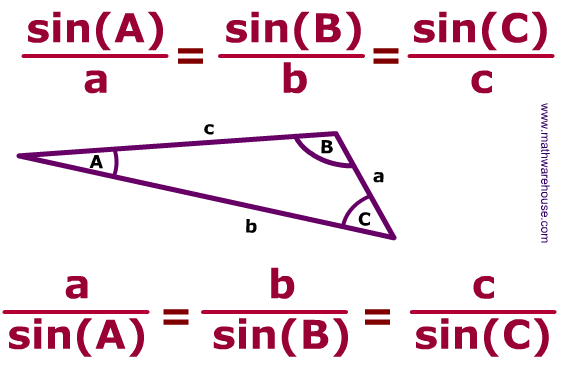Application of Sine Rule

• Sine rule can be used to find the unknown side or length of a triangle.
• Sine rule can also be used to find the unknown angle of a triangle.

To find the unknown side or length of a triangle, three essential parameters must be provided and these parameters are:

• The angle opposite to the unknown side or length of the triangle.
• The length of a side of the triangle (b).
• The angle opposite to the length of side (b) of the triangle.

Let’s take for example we have a triangle and we want to determine the unknown side of this triangle, when the opposite angle is given, another length of the side of the triangle and its opposite angle is also given. Like the image below.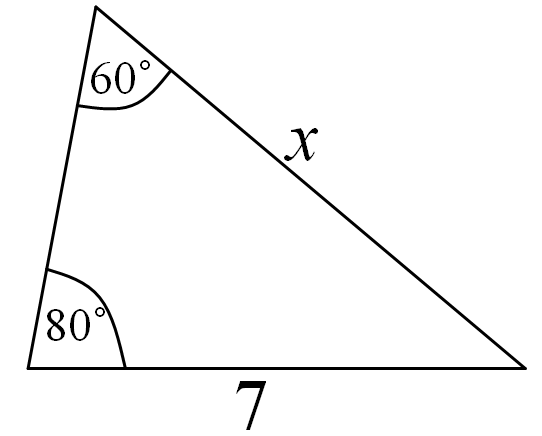From the image above, one can see that;
The angle opposite to the unknown length of the side of the triangle is 80°. (A)
The length of the side of the triangle given is 7. (b)
The angle opposite to the length of the side of the triangle given is 60° (B)
The unknown length of the side of the triangle is x. (a)

Applying the sine rule to find the value of x:

a / sin(A) = b / sin(B)

x / sin(80°) = 7 / sin(60°)
x / 0.9848 = 7 / 0.8660

Applying cross multiplication

x (0.8660) = 7 (0.9848)
x (0.8660) = 6.8936

Dividing both sides by 0.8660

x = 6.8936 / 0.8660
x = 7.96

Therefore, the unknown length of the side of the triangle, x is 7.96.

## How to Calculate and Solve for the Width, Height, Length and Area of a Rectangular Prism | The Calculator Encyclopedia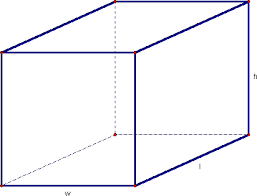The image above is a rectangular prism.

To compute the area of a rectangular prism requires three essential parameters which are the length, width and height of the rectangular prism.

A = 2wl + 2hl + 2hw

Where;
A = Area of the rectangular prism
l = Length of the rectangular prism
w = Width of the rectangular prism
h = Height of the rectangular prism

Let’s solve an example;
Find the area of a rectangular prism with a width of 10 cm, a height of 17 cm and a length of 14 cm.

This implies that;
l = Length of the rectangular prism = 14 cm
w = Width of the rectangular prism = 10 cm
h = Height of the rectangular prism = 17 cm

A = 2wl + 2hl + 2hw
A = 2 x 10 x 14 + 2 x 17 x 14 + 2 x 17 x 10
A = 280 + 476 + 340
A = 1096

Therefore, the area of the rectangular prism is 1096 cm2.

Calculating the Length of a Rectangular Prism using the Area, Width and Height of the Rectangular Prism.

l = A – 2hw / 2 (w + h)

Where;
l = Length of the rectangular prism
A = Area of the rectangular prism
w = Width of the rectangular prism
h = Height of the rectangular prism

Let’s solve an example;
Find the length of a rectangular prism with a width of 9 cm, a height of 12 cm and a Area of 250 cm2.

This implies that;
w = Width of the rectangular prism = 9 cm
h = Height of the rectangular prism = 12 cm
A = Area of the rectangular prism = 250 cm2

l = A – 2hw / 2 (w + h)
l = 250 – 2 x 12 x 9 / 2 (9 + 12)
l = 250 – 216 / 2 (21)
l = 34 / 42
l = 0.81

Therefore, the length of the rectangular prism is 0.81 cm.

## How to Calculate and Solve for the Width, Height, Length and Volume of a Rectangular Prism | Nickzom CalculatorThe image above is a rectangular prism.

To compute the volume of a rectangular prism requires three essential parameters which are the length, width and height of the rectangular prism.

V = lwh

Where;
V = Volume of the rectangular prism
l = Length of the rectangular prism
w = Width of the rectangular prism
h = Height of the rectangular prism

Let’s solve an example;
Find the volume of a rectangular prism with a length of 18 cm, a width of 12 cm and a height of 21 cm.

This implies that;
l = Length of the rectangular prism = 18 cm
w = Width of the rectangular prism = 12 cm
h = Height of the rectangular prism = 21 cm

V = lwh
V = 18 x 12 x 21
V = 4536

Therefore, the volume of the rectangular prism is 4536 cm3.

Calculating the Length of a Rectangular Prism using the Volume, Width and Height of the Rectangular Prism.

l = V / wh

Where;
l = Length of the rectangular prism
V = Volume of the rectangular prism
w = Width of the rectangular prism
h = Height of the rectangular prism

Let’s solve an example;
Find the length of a rectangular prism with a width of 19 cm, a height of 24 cm and a Volume of 250 cm3.

This implies that;
w = Width of the rectangular prism = 19 cm
h = Height of the rectangular prism = 24 cm
V = Volume of the rectangular prism = 250 cm3

l = V / wh
l = 250 / 19 x 24
l = 250 / 456
l = 0.55

Therefore, the length of the rectangular prism is 0.55 cm.

## How to Calculate and Solve for the Angle, Radius and Length of an Arc of a Circle | The Calculator Encyclopedia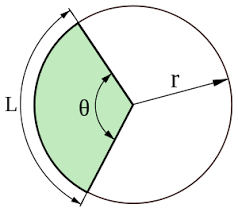The image above represents the length of an arc of a circle.

To compute the length of an arc of a circle, two essential parameters are needed and this parameters are radius of the circle (r) and angle of the circle (α).

The formula for calculating the length of an arc of a circle:

L = απr / 180

Where;
L = Length of an arc of the circle
α = Angle of the circle
r = radius of the circle

Let’s solve an example:
Find the length of an arc of a circle when the angle of the circle is 90° and the radius of the circle is 20 cm.

This implies that;
α = Angle of the circle = 90°
r = Radius of the circle = 20 cm

L = απr / 180
L = 90 x 3.142 x 20 / 180
L = 5655.6 / 180
L = 31.42

Therefore, the length of an arc of the circle is 31.42 cm.

Calculating the Angle of a Circle using the Radius of the Circle and Length of an Arc of the Circle.

α = 180L / πr

Where;
L = Length of an arc of the circle
r = Radius of the circle

Let’s solve an example;
Find the angle of the circle when the length of an arc of the circle is 60° and a radius of 140 cm.

This implies that;
L = Length of an arc of the circle = 60°
r = Radius of the circle = 140 cm

α = 180L / πr
α = 180 x 60 / 3.142 x 140
α = 10800 / 439.88
a = 24.55

Therefore, the angle of the circle is 24.55°.

## How to Calculate and Solve for the Length and Area of a Pentagon | Nickzom Calculator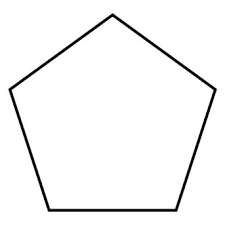The image above is a pentagon.

To compute the area of a pentagon, one essential parameter is needed and this parameter is length of side (a).

The formula for calculating the area of a pentagon:

A = a2(5(5 + 2√5) / 4

Where;
A = Area of the pentagon
a = length of side

Let’s solve an example;
Find the area of the pentagon when the length of side is 30 cm.

This implies that;
a = length of side = 30 cm

A = a2(5(5 + 2√5) / 4
A = 302(5(5 + 4.47) / 4
A = 900√(5(9.47) / 4
A = 900√47.36 / 4
A = 900√11.84
A = 900 x 3.44
A = 3096

Therefore, the area of the pentagon is 3096 cm2.

Calculating the length of side using the area of the pentagon.

a = √(4A / √5(5 + 2√5))

Where;
a = length of side
A = Area of the pentagon

Let’s solve an example;
Find the length of side with an area of 150 cm2.

This implies that;
A = Area of the pentagon = 150 cm2

a = √(4A / √5(5 + 2√5))
a = √(4 x 150 / √5(5 + 1.148))
a = √(600 / √5(6.148))
a = √(600 / √30.74)
a = √(600 / 5.54)
a = √108.30
a = 10.41

Therefore, the length of side is 10.41 cm.

## How to Calculate the Volume, Length, Width and Height of a Cuboid | Nickzom Calculator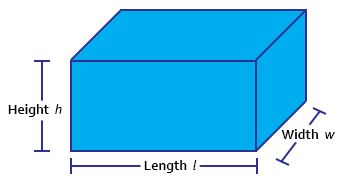The image above represents a cuboid.
To compute the volume of a cuboid requires three essential parameters which are the length, width and height of the cuboid.

The formula for computing the volume of a cuboid is:

V = lwh

Where:
V = Volume of the Cuboid
l = Length of the Cuboid
w = Width of the Cuboid
h = Height of the Cuboid

Let’s solve an example
Find the volume of a cuboid with a length of 6 cm, width of 2 cm and a height of 10 cm.

This implies that:
l = length of the cuboid = 6
w = width of the cuboid = 2
h= = height of the cuboid = 10

V = lwh
V = 6 x 2 x 10
V = 120

Therefore, the volume of the cuboid is 120 cm3.

Calculating the Length of a cuboid when Volume, Width and Height are Given

The formula is l = V / (w)(h)

Where;
V = Volume of the Cuboid
l = Length of the Cuboid
w = Width of the Cuboid
h = Height of the Cuboid

Let’s solve an example:
Find the length of a cuboid with a volume of 440 cm3 , a width of 5 cm and a height of 11 cm.

This implies that;
V = Volume of the cuboid = 440 cm3
w = width of the cuboid = 5 cm
h  = height of the cuboid = 11 cm

l =  V / (w)(h)
l = 440 / (5)(11)
l = 440 / 55
l = 8 cm

Therefore, the length of the cuboid is 8 cm.

Calculating the Width of a cuboid when Volume, Length and Height are Given

The formula is w = V / (l)(h)

Where;
V = Volume of the Cuboid
l = Length of the Cuboid
w = Width of the Cuboid
h = Height of the Cuboid

Let’s solve an example:
Find the width of a cuboid with a volume of  180 cm3 , a length of 6 cm and a height of 10 cm

This implies that;
V = Volume of the cuboid = 180 cm3
l = length of the cuboid = 6 cm
h  = height of the cuboid = 10 cm

w =  V / (l)(h)
w = 180 / (6)(10)
w = 180 / 60
w = 3 cm

Therefore, the width of the cuboid is 3 cm.

Calculating the Height of a cuboid when Volume, Length and Width are Given

The formula is h = V / (l)(w)

Where;
V = Volume of the Cuboid
l = Length of the Cuboid
w = Width of the Cuboid
h = Height of the Cuboid

Let’s solve an example:
Find the height of a cuboid with a volume of 195 cm3 , a length of 5 cm and a width of 3 cm

This implies that;
V = Volume of the cuboid = 195 cm3
l = length of the cuboid = 5 cm
w  = width of the cuboid = 3 cm

h =  V / (l)(w)
h = 195 / (5)(3)
h = 195 / 15
h = 13 cm

Therefore, the height of the cuboid is 13 cm.

## How to Calculate and Solve for the Length and Perimeter of a Rhombus | Nickzom Calculator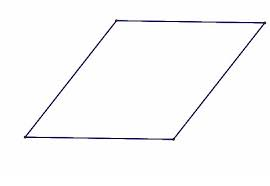The image above is a rhombus.

To compute the area of a rhombus, one essential parameter is needed and this parameter is length of side (a).

The formula for calculating the perimeter of a rhombus:

P = 4a

Where;
P = Perimeter of the rhombus
a = length of side

Let’s solve an example;
Find the perimeter of a rhombus when the length of side is 30 cm.

This implies that;
a = Length of side = 30 cm

P = 4a
P = 4 x 30
P = 120

Therefore, the perimeter of the rhombus is 120 cm.

Calculating the Length of Side using the Perimeter of the Rhombus.

a = P / 4

Where;
a = Length of Side
P = Perimeter of the rhombus

Let’s solve an example;
Given that the perimeter of the rhombus is 180 cm. Find the length of side?

a = P / 4

This implies that;
P = perimeter of the rhombus = 180 cm

a = P / 4
a = 180 / 4
a = 45

Therefore, the length of side is 45 cm.